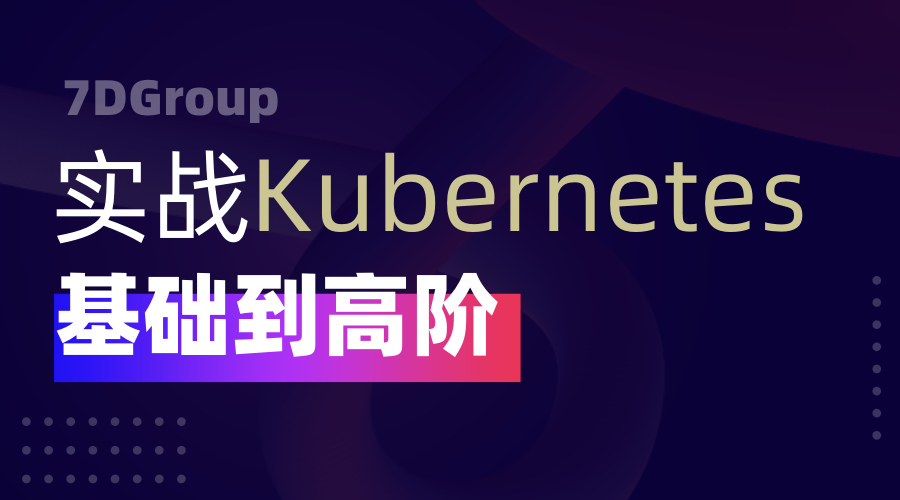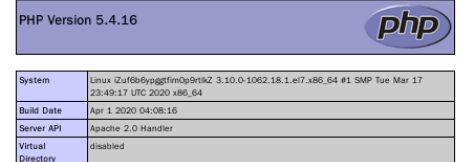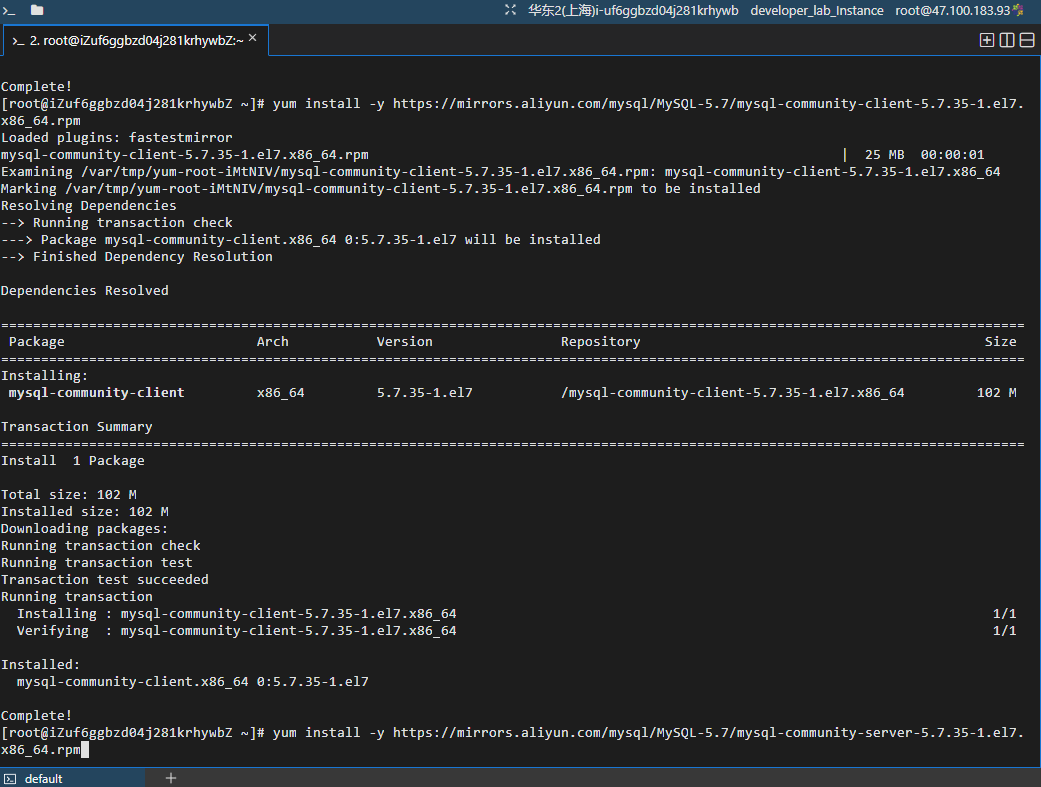Spark之BloomFilter有趣的bitwise运算

+关注继续查看

BitArray巧妙的核心设计
BitArray内部采用long[] data来表示一个大的bitmap，long类型相比int在相同的数组个数下可以存放更多的bit信息。

// 将指定index位置的bit位设置为1，表示指定的index处有值
void set(long index) {
data[(int) (index >>> 6)] |= (1L << index);
}

jshell> -2 >> 1
$1 ==> -1 jshell> -2 >>> 1$2 ==> 2147483647

Java中一个long类型占8个byte，也就是64个bit，如果让我们实现给定一个index，判断该index属于数组的第几个元素，我们可能会这么实现：

data[index / 64]

BitArray中让人懵逼的是直接对一个long类型的值进行了1L << index操作，如果让我实现的话，我会这么做：1 << (index % 64)，我们知道long只有64位，如果index大于64位呢？

jshell> 1L << 65
\$1 ==> 2

If the promoted type of the left-hand operand is long, then only the six lowest-order bits of the right-hand operand are used as the shift distance. It is as if the right-hand operand were subjected to a bitwise logical AND operator & (§15.22.1) with the mask value 0x3f (0b111111). The shift distance actually used is therefore always in the range 0 to 63, inclusive.

1L << index 相当于
1L << (index & 0x3f) 相当于
1L << (index & 0b111111) 相当于
1L << (index % 2^6) 相当于
1L << (index % 64)

void set(long index) {
data[(int) (index >>> 6)] |= (1L << index);
}

// 等价于
void set(long index) {
data[index / 64] |= (1L << (index % 64));
}

a & 1 = 0 // 偶数
a & 1 = 1 // 奇数

a % (2^n) 等价于 a & (2^n - 1)

a * (2^n) 等价于 a << n

a / (2^n) 等价于 a >> n

x的相反数为~x + 1
// 原理上和计算机采用二进制补码（2's complement）的表示形式有关

if (x == a):
x = b
else:
x = a

Spark内部除了BloomFilter在用MurmurHash以外，UnsafeRow在计算Hash时，也有用到。Kubernetes + Spring Cloud 集成链路追踪 SkyWalking

4 09 0《冬季实战营第一期：从零到一上手玩转云服务器》实践报告

10 010 08 006_spring_ 依赖注入| 学习笔记

11 0【冬季实战营第一期：从零到一上手玩转云服务器】学习报告

8 005_spring_ 配置文件| 学习笔记

6 017 0
765

0

《2021云上架构与运维峰会演讲合集》

《零基础CSS入门教程》

《零基础HTML入门教程》